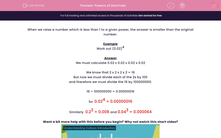# Powers of Decimals

In this worksheet, students raise simple decimal numbers to the given powers.Key stage:  KS 2

Curriculum topic:   Maths and Numerical Reasoning

Curriculum subtopic:   Decimals

Difficulty level:#### Worksheet Overview

When we raise a number which is less than 1 to a given power, the answer is smaller than the original number.

Example

Work out (0.02)4

We must calculate 0.02 x 0.02 x 0.02 x 0.02

We know that 2 x 2 x 2 x 2 = 16

But now we must divide each of the 2s by 100

and therefore we must divide the 16 by 100000000.

16 ÷ 100000000 = 0.00000016

So  0.024 = 0.00000016

Similarly  0.23 = 0.008 and 0.043 = 0.000064

Want a bit more help with this before you begin? Why not watch this short video?

### What is EdPlace?

We're your National Curriculum aligned online education content provider helping each child succeed in English, maths and science from year 1 to GCSE. With an EdPlace account you’ll be able to track and measure progress, helping each child achieve their best. We build confidence and attainment by personalising each child’s learning at a level that suits them.

Get started